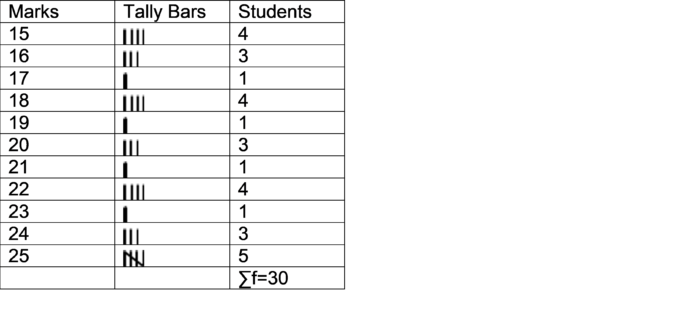# T.R. Jain and V.K. Ohri Solutions Class 11 Statistics Economics Chapter 4 - Organisation of Data

T.R. Jain and V.K. Ohri Solutions for Class 11 Statistics for Economics Chapter 4 – Organisation of Data is regarded as an important concept to be studied thoroughly by the students. Here, we have provided T.R. Jain and V.K. Ohri Solutions for Class 11.

 Board CBSE Class Class 11 Subject Statistics for Economics Chapter Chapter 4 Chapter Name Organisation of Data Number of questions solved 02 Category T.R. Jain and V.K. Ohri

Chapter 4: Organisation of data covers the following mentioned concepts:

• What is the classification of data?
• Concept of variable
• Conversion of raw data into statistical series
• Types of frequency distribution

## T.R. Jain and V.K. Ohri Solutions for Class 11 Statistics for Economics Chapter 4 – Organisation of Data

Question 1

Convert the following cumulative frequency series into a simple frequency series:

• 4 students obtained less than 10 marks
• 20 students obtained less than 20 marks
• 40 students obtained less than 30 marks
• 48 students obtained less than 40 marks
• 50 students obtained less than 50 marks

Solution:

Conversion of cumulative frequency series into a simple frequency series

 Cumulative Frequency Series Simple Frequency Series Marks less than Number of students Marks Number of students 10 4 0-10 4 20 20 10-20 20-4 = 16 30 40 20-30 40-20 = 20 40 48 30-40 48-40 = 8 50 50 40-50 50-48 = 2

Question 2

30 Students of Class XI obtained following marks in economics. Classify the data in the form of individual series, discrete series, continuous series and cumulative frequency series.

 24 16 22 20 16 18 19 17 25 18 24 20 16 15 22 20 21 23 18 22 25 22 15 24 15 25 18 25 15 20

Solution:

Individual series:

 15 15 15 15 16 16 16 17 18 18 18 18 19 20 20 20 21 22 22 22 22 23 24 24 24 25 25 25 25 25

Discrete Series:Continuous Series:Cumulative frequency series:

 Marks No. of Students Marks No. of Students Less than 15 0 More than 12 30 Less than 19 9 + 4 =13 More than 16 30 – 4 =26 Less than 23 13 + 9 =22 More than 20 26 – 9 =17 Less than 27 22 + 8 = 30 More than 24 17 – 9 =8

Also Check: What is Classification of data?

These solutions are considered to be the best solutions for the chapter. Stay tuned for questions papers, sample papers, syllabus, and relevant notifications on our website